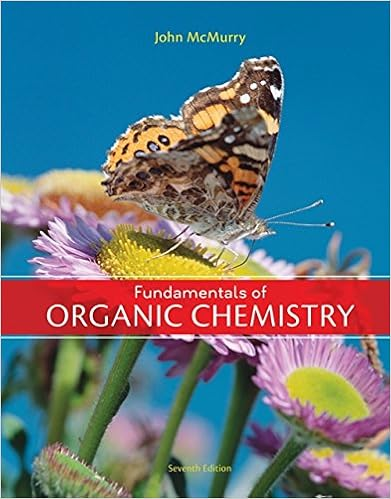# 26 given for which of the following are able to

• Notes
• 14
• 75% (4) 3 out of 4 people found this document helpful

This preview shows page 3 - 4 out of 14 pages.

##### We have textbook solutions for you!
The document you are viewing contains questions related to this textbook.The document you are viewing contains questions related to this textbook.
Chapter 9 / Exercise 9.21
Fundamentals of Organic Chemistry
McMurryExpert Verified
____26.Given for , which of the following are able to oxidize I.II.III.IV.?a.I and IId.I, III and IVb.II and IIIe.I, II and IIIc.I and IV
____27.An electrochemical cell consists of a chromium electrode in 1.0 mol/L solution of (chromium nitrate), a silver electrode in 1.0 mol/L solution of (silver nitrate) and a salt bridge. The spontaneous cell reaction isWhen the two electrodes are connected by a wire, which of the following does nottake place?
____28.The function of the salt bridge in a galvanic cell is
Short Answer29.What would be the shape of NO3-1? Explain your reasoning.30.Why do metals conduct electricity?31.Differentiate between an effective collision and an ineffective collision.32.How is a reaction mechanism designed?33.If the pH of an acid solution at 25oC is 6.18, what is the pOH; and the [H1+], [OH1-] in mol/L?34.If the [H1+] of a solution at 25oC is 1.7  10-4mol/L, calculate the [OH1-] in mol/L, the pH, and the pOH.35.Calculate for the following equation:Decide whether the equation represents a spontaneous or a nonspontaneous reaction at standard conditions.36.What process occurs at the cathode of an electrolytic cell while the cell is operating?37.Cubaneis the name given to a newly synthesized alkane which has the formula C8H8. This is a three-dimensional carbon-carbon molecule where every carbon atom is bonded to three carbon atoms plus one hydrogen atom. Sketch the structure andexplain why it tends to be unstable.38.Write the reaction showing how the ester., can be prepared from a carboxylic acid and an alcohol. Provide the names of all reactants below your equation.39.The structure given below is a polymer classified as a polyamide. This polymer is stable in temperatures above 350°C, and can be used as a protective coating on hot surfaces. What monomers were used to make this polyamide?40.Explain why CH3F is a polar molecule while CF4is not.41.Is ammonia a polar molecule? Explain your answer using a diagram.42.Predict the shape and bond angles of hydrogen sulphide, H2S.43.Explain why 44.How does the rate of change of the product compare to the rate of change of the reactant?45.Describe how the chemical nature of the reactants can control the rate of the reaction. Give an example.46.How can the.
##### We have textbook solutions for you!
The document you are viewing contains questions related to this textbook.The document you are viewing contains questions related to this textbook.
Chapter 9 / Exercise 9.21
Fundamentals of Organic Chemistry
McMurryExpert Verified
•••